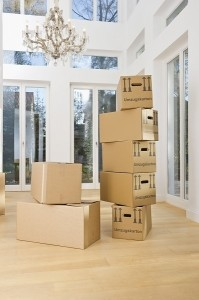# Dimensions of a Box

Here are dimensions of a box that is used for different purposes. Considered are a square and a rectangular box with their box measurements.

### Square Box

A square box has equal sides. The length, height, and width are of the same dimensions. This means the top, bottom, and 4 sides should all measure the same. However, there is a variation here. A square box can also have smaller side measurements. For instance, consider these dimensions of a box: top and bottom length and width measure 24 inches each, while the 4 lateral sides measure 5 inches in width (or height) and 24 inches in length. These box measurements define a flat box.

The flat square box above has a volume of 2,880 square inches or 1.7 square feet. A perfect square box, measuring 24 inches in length, width, and height, has a 13,824 square-inch volume or 8 square feet. The dimensions of a box that is square shaped vary depending on the measurements of its lateral sides.

###Rectangular Box

The dimensions of a box with a rectangular shape have the length and width differently sized. The lateral sides do not affect its shape like the width and length do. In fact, box measurements defining a box square or rectangle depends on its width and length dimensions. To convert the above perfect square box (with length, width, and sides all measuring 24 inches) into a rectangle, either the length or the width should be changed. An increase or decrease in either of them would do the trick.

Thus, a box measuring 24 inches by 15 inches with a height of 24 inches is a rectangular box, despite the minimal difference of 5 inches. From a distance, this rectangle may not seem different from a perfect box. Nonetheless, by mathematical definition, they are differently shaped.

### Thick Boxes

With thick boxes, there is a difference between the outer and inner dimensions of a box. For instance, a box measuring 1 by 1 foot, with a height of also 1 foot but with a thickness of 1 inch will have different exterior and interior measurements. Just subtract 1 inch from the dimensions and one easily gets the inner box measurements. In this case, it is 11 by 11 by 11 inches, or 1331 square inches in volume.

This difference in exterior and interior sizes is only applicable to thick boxes, like those made of wood or thick metal. With thin cardboard or illustration board materials, thickness is negligible in the dimensions of a box.